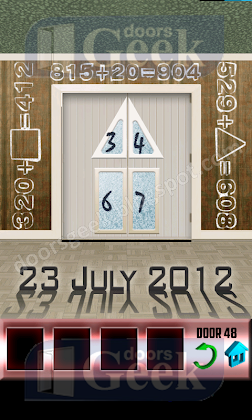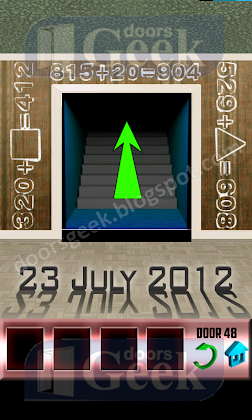## Monday, April 22, 2013

### 100 Doors Level 48 Walkthrough1. The 23 July 2012 is the clue. Near the door you have some numbers, you must think of them like dates. So we have above the door a equation that is already resolved: 815+20=904, think of this like 8(August) 15(day) +20 days = 9(September) 04(day) Now you have to solve the other equations: On the left we have: 320+square=412 this can be translated in: March 20 + X = April 12 and x=23(days) On the right side we have: 529+triangle=608 this gives the following dates: May 29 + X = June 08 so x=10(days) So the solution is top row 10 bottom row 23.2. Now you can play the next level.